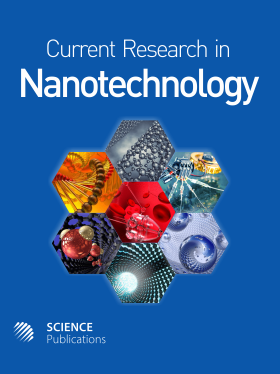Frequency: Continuous
ISSN: 1949-0216 (Print)
ISSN: 1949-0224 (Online)
Research Article Open Access

# Modeling of Piezoelectric Actuators Based on Bayesian Regularization Back Propagation Neural Network

Wen Wang1, Zhu Zhu1, Yanding Wei1 and Zichen Chen1
• 1 Zhejiang University, China

## Abstract

Problem statement: Piezoelectric actuator is a kind of key driving components for micropositioning stages, micropumps, micro valves, micro gripper and so on in the fields of micro/nano technology such as integrated circuit manufacturing, precision instruments, ultra precision fabrication, biomedical manipulation. It has lots of advantages including high stiffness, fast response times, less heat generating, low power consumption and large force output. But the hysteresis nonlinearity seriously affects working performance of actuators. So a lot of models were proposed to describe the hysteresis nonlinearity. A popular model which was widely used is the Preisach model. In order to obtain accurate displacement output corresponding to arbitrary input voltage with the Preisach model, function output approximation is needed. Approach: In this study, firstly the Preisach model was introduced. Then the function modeling of Preisach model based on a Bayesian Regularization Back Propagation Neural (BRBPNN) was presented and a three layers BPNN was designed. Finally, the BRBPNN was trained in Neural Network toolbox of MATLAB6.0. The Preisach function values not at equal diversion points were calculated by the trained network and the actual displacement outputs and theoretical values corresponding to random voltages input were compared. Results: Experimental results indicate that theoretical displacements and measured displacements agree with very well, the maximum displacement error is 0.35μm and the standard deviation is 0.24 μm. Conclusion: The BRBPNN could realize function approximation in Preisach modeling accurately and could meet the precision requirement in the field of modeling and controlling of piezoelectric actuators.

Current Research in Nanotechnology
Volume 1 No. 1, 2010, 1-6

DOI:

Published On: 15 June 2011

How to Cite: Wang, W., Zhu, Z., Wei, Y. & Chen, Z. (2010). Modeling of Piezoelectric Actuators Based on Bayesian Regularization Back Propagation Neural Network. Current Research in Nanotechnology, 1(1), 1-6. https://doi.org/10.3844/ajnsp.2010.1.6

• 2,490 Views